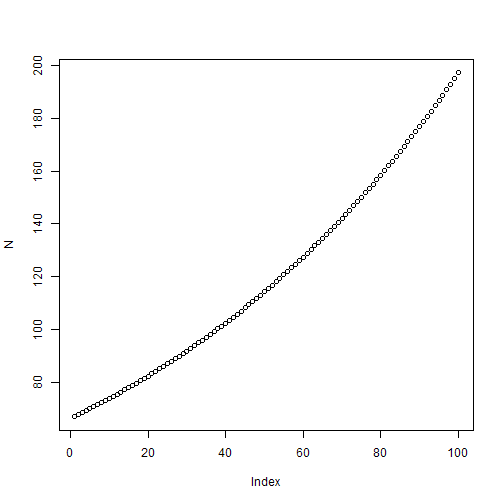``````pm <- read.csv(file = "c:\\R\\data\\dapengde_DummyR_PM25.csv")
par(mfrow=c(1， 3))
plot(pm[, 1], pm[, 2], cex = 2, type = "l") # 第一张图
plot(pm[, 1], pm[, 3], cex = 2, type = "l") # 第二张图
plot(pm[, 1], pm[, 4], cex = 2, type = "l") # 第三张图
``````

``````par(mfrow=c(1,3))
for (i in c(2, 3, 4)) plot(pm[, 1], pm[, i], cex = 2, type = "l") # 让 i 的取值在2, 3, 4这三个数中转一圈，做第 i 张图。
``````

`i` 转的那个圈，可以是数字，也可以是别的，比如字符串：

``````for (i in c("Good", "Morning", "!")) print(i)
``````
``````练习05.1 用`print()` 指令打印 1 到 100 之间的奇数。
``````

R 中常用的循环语句，除了 `for()` 之外，还有`while()``until()`，相互之间可以换用。

``````# 方法 1：每年分别计算，分别存储人口数。
N1 <- 66.8
r <- 0.011
N2 <- N1 + r * N1
N3 <- N2 + r * N2  # 如此一直写到 N100。

# 方法 2：每年分别计算，用一个向量来存储各年人口总数。
N <- numeric(100)
N <- 66.8
N <- N + r * N
N <- N + r * N  # 如此一直写到 N。

# 方法 3： 用循环语句计算，用一个向量来存储各年人口数。
N <- numeric(100)
N <- 66.8
for (t in 1:99) N[t + 1] <- N[t] + r * N[t]  # 不必一直写到N100。
plot(N)
````````````for(t in 1:99)
{
N[t+1] <- N[t] + r * N[t]
print(t + 2007)
print(N[t+1])
}
``````

• 圆括号 ()。用作命令和数学表达式，如 `mean(x), (1 + 2) * 3`
• 方括号 []。用作下标，调用向量中的某个元素，如`x, pm[2,6]`
• 花括号 {}。用作编程，把一组指令组合在一起，如`for () {}`
``````练习05.2 生成一个矩阵 m （方法见 matrix 函数的帮助信息），使得 m[i,j] = x[i] * y[j]， 其中 x <- c(2, 3, 5)， y <- c(1, 2, 3, 4)。
``````

## 有用的信息：

( 连载中，待续 )

### 16 Responses to “R 菜鸟入门篇 第05篇 循环”

1. 只擅长运动和小炒的男淫路过。
单次分析或者画图对于R来说，速度上是没有优势，拼的就是apm了

• 是啊，无奈生活经常是一次又一次的循环往复，日出日落，月圆月缺，年尾年头…….

2. 男人至少要擅长一项运动,一种乐器,一种编程….和拿手的几个小炒.

悲催，我一个都不会

• 没有最擅长，只有更擅长。调侃而已，不必当真嘛。

3. 最后的小练习挺有意思的，不知道@dapeng还有没有别的练习题了？
看了 R in Action 的前六章，感觉什么都会一点了，但是一到自己的数据上就麻爪。

• 这个R菜鸟入门的重点就是练习，跟着做一遍就能处理自己的数据了。

4. 求教：我的R code如下：
for (i in c(1:7)) for (j in c(“wind”,”sorlar radiation”,”CO”,”NO”,”NO2″,”O3″,”HC”))plot(hw116[,i],ylab=(j))
问题是R只显示最后一张HC图，前面6张就不显示了，请问怎么能让R显示前面6张？

• 后面的把前面的覆盖了。需要事先设置好页面布局，分成几行几列，用par()或layout()都可以。

5. 大鹏：
你好，这个怎么写呢？我刚开始学R，谢谢哈！
生成一个矩阵 m （方法见 matrix 函数的帮助信息），使得 m[i,j] = x[i] * y[j]， 其中 x <- c(2, 3, 5)， y <- c(1, 2, 3, 4)。

• 我也不知道有没有现成的函数。最笨的方法，就是用个for循环吧。

6. 大鹏，我也用R做过各省GDP地图，但有几个问题想和你探讨一下：
一，和ArcGIS相比，总感觉R的色调等不是很上档次，
二，如果要把31省份合并成七大区或东中西三大区，用R的话各区边界就乱套了。
三，无法在地图上加上柱状图（ArcGIS可以）

以上三点你有什么好的解决方案吗？

• R和ArcGIS比赛绘制地图的话，就好比铁人三项选手挑战游泳专业选手，R显得业余，毕竟它不是专门干这个的。R的优势在于免费和灵活。一方面，如果R能解决的GIS问题，就不必花钱购买昂贵的ArcGIS了（当然，盗版软件是另外一个话题了，不提）；另一方面，R在编程上具有优势，如果结合现成的包来对数据进一步分析，那么是非常让人心动的。R的色调也是一门学问，怎么样好看全靠用户自己琢磨了。至于省份的合并和柱状图，我没有试过，但是这应该算不上高端功能，R应该能胜任的。
用R处理GIS数据做地图我也很陌生，功夫粗浅得很，只是知道能实现，而且本文是个R入门介绍而已。用R处理GIS数据这种高大上的东西，还是期待你们发烧友多折腾。我已经老了，过了折腾这个的岁数了……

7. 大鹏，第五章开始，练习题我就有做不出的了，列出1-100的奇数这个，我就写不出来，我只能照猫画虎，滑到这一步（如下），该怎么写呢？
x <- 1:100
x
for (i in c(1:100))
print(i)

• print(seq(1,100,2))

8. x <- c(2, 3, 5)
y <- c(1, 2, 3, 4)

for (t in 1:3)print(x[t]*y)

接下来就不知道该咋办了

9. 不用for循环好像也可以：

x <- matrix(c(2,3,5), nrow=3)
y <- matrix(c(1,2,3,4), ncol=4)
m <- matrix (x%*%y, nrow=3, ncol=4)

不知道这样对不对 …